# Solution of triangles Questions and Answers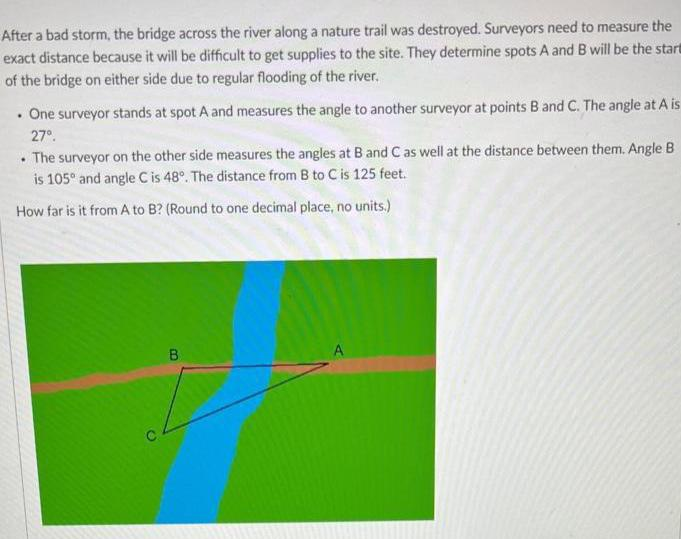Geometry
Solution of triangles
After a bad storm, the bridge across the river along a nature trail was destroyed. Surveyors need to measure the exact distance because it will be difficult to get supplies to the site. They determine spots A and B will be the start of the bridge on either side due to regular flooding of the river. . One surveyor stands at spot A and measures the angle to another surveyor at points B and C. The angle at A is 27º. . The surveyor on the other side measures the angles at B and C as well at the distance between them. Angle B is 105° and angle C is 48°. The distance from B to C is 125 feet. How far is it from A to B? (Round to one decimal place, no units.)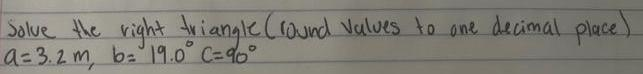Geometry
Solution of triangles
Solve the right triangle (round values to one decimal place) a=3.2 m, b= 19.0⁰° C = 90°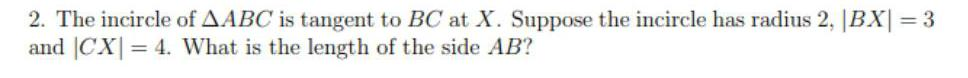Geometry
Solution of triangles
The incircle of AABC is tangent to BC at X. Suppose the incircle has radius 2, |BX|=3 and |CX| = 4. What is the length of the side AB?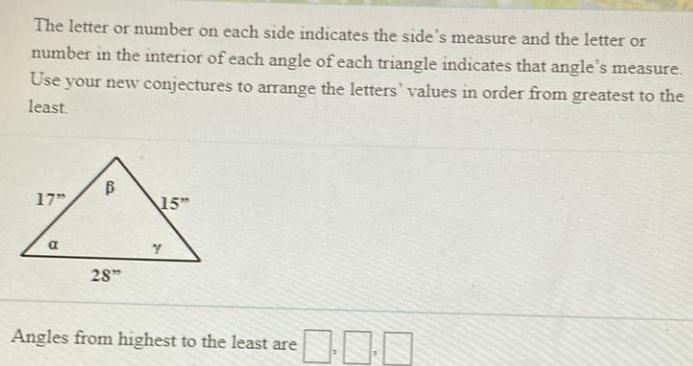Geometry
Solution of triangles
The letter or number on each side indicates the side's measure and the letter or number in the interior of each angle of each triangle indicates that angle's measure. Use your new conjectures to arrange the letters' values in order from greatest to the least. Angles from highest to the least are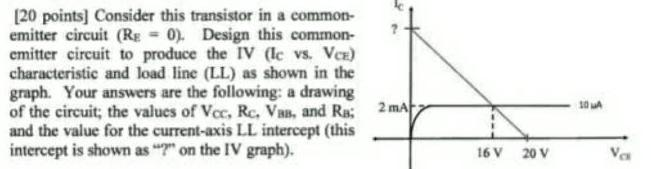Geometry
Solution of triangles
Consider this transistor in a common-emitter circuit (RE = 0). Design this common- emitter circuit to produce the IV (lc vs. VCE) characteristic and load line (LL) as shown in the graph. Your answers are the following: a drawing of the circuit; the values of Vcc, Rc, Van, and Ra; and the value for the current-axis LL intercept (this intercept is shown as "?" on the IV graph).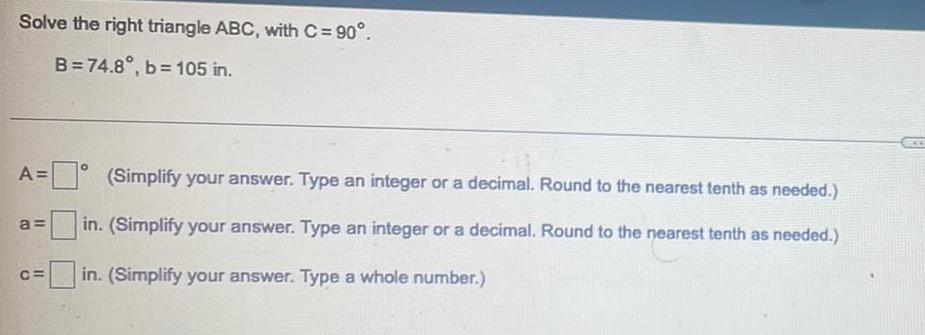Geometry
Solution of triangles
Solve the right triangle ABC, with C = 90°. B=74.8°, b= 105 in. A=___(Simplify your answer. Type an integer or a decimal. Round to the nearest tenth as needed.) a=__(Simplify your answer. Type an integer or a decimal. Round to the nearest tenth as needed.) C=___(Simplify your answer. Type an integer or a decimal. Round to the nearest tenth as needed.)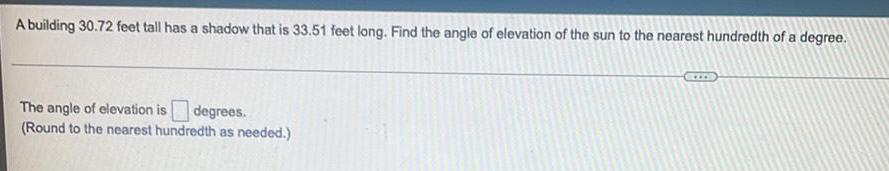Geometry
Solution of triangles
A building 30.72 feet tall has a shadow that is 33.51 feet long. Find the angle of elevation of the sun to the nearest hundredth of a degree. The angle of elevation is degrees. (Round to the nearest hundredth as needed.)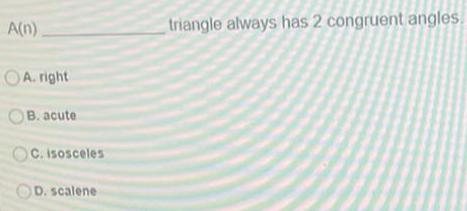Geometry
Solution of triangles
A(n)___triangle always has 2 congruent angles. A. right B. acute C. isosceles D. scalene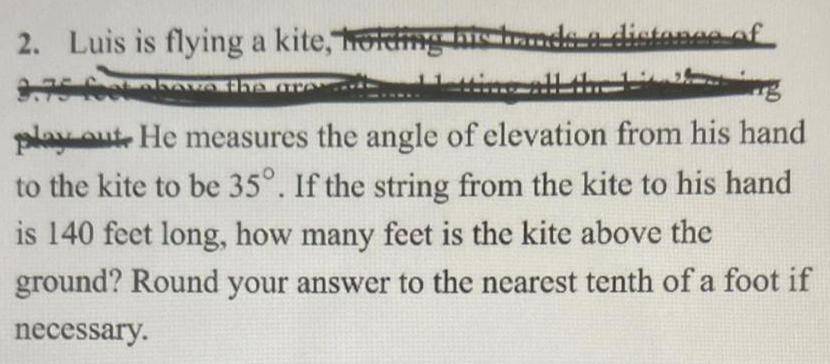Geometry
Solution of triangles
Luis is flying a kite, holding his hands a distance of 9.75 feet above the ground play out. He measures the angle of elevation from his hand to the kite to be 35°. If the string from the kite to his hand is 140 feet long, how many feet is the kite above the ground? Round your answer to the nearest tenth of a foot if necessary.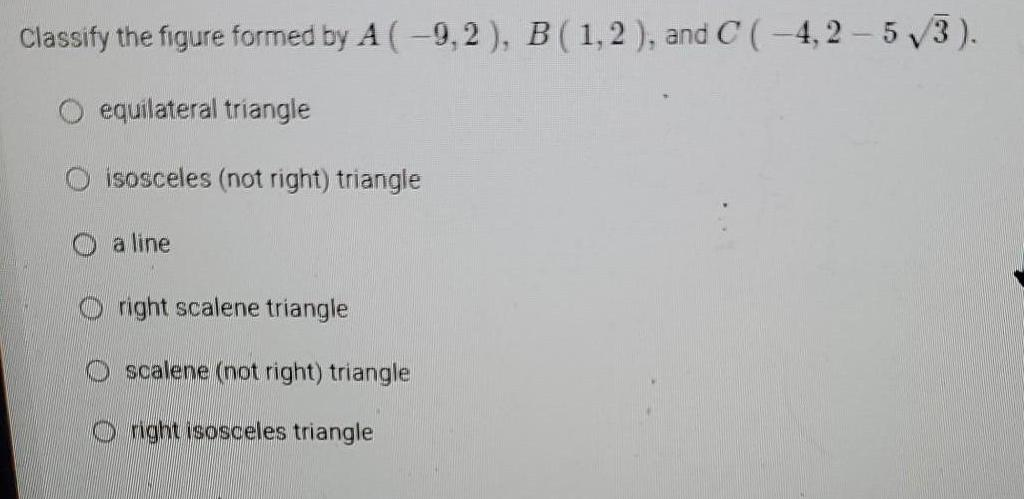Geometry
Solution of triangles
Classify the figure formed by A (-9,2), B (1,2), and C (-4,2-5√3). equilateral triangle isosceles (not right) triangle a line right scalene triangle scalene (not right) triangle right isosceles triangle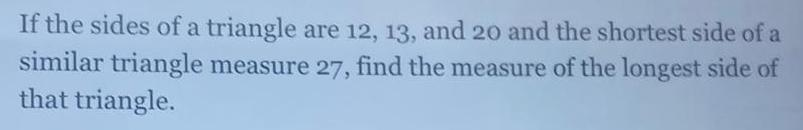Geometry
Solution of triangles
If the sides of a triangle are 12, 13, and 20 and the shortest side of a similar triangle measure 27, find the measure of the longest side of that triangle.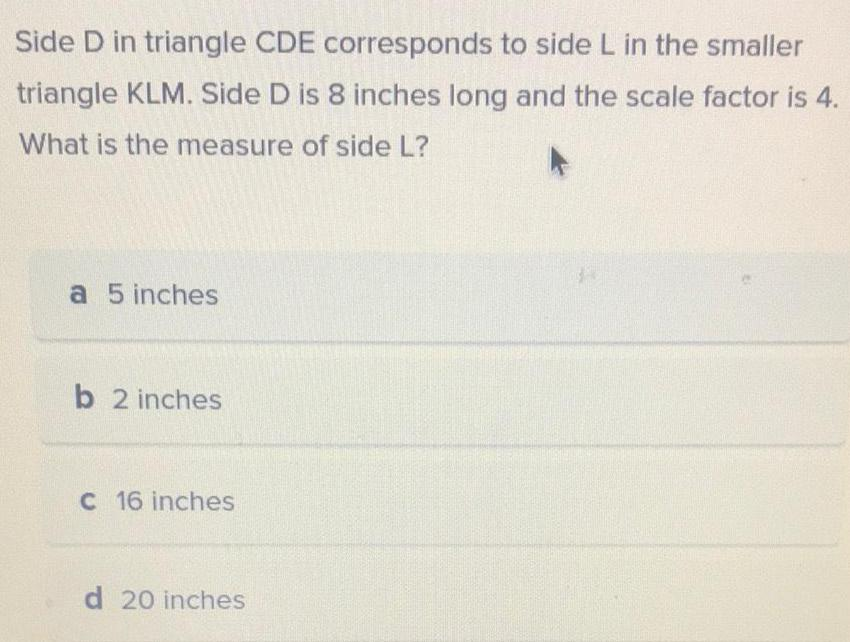Geometry
Solution of triangles
Side D in triangle CDE corresponds to side L in the smaller triangle KLM. Side D is 8 inches long and the scale factor is 4. What is the measure of side L? a 5 inches b 2 inches c 16 inches d 20 inches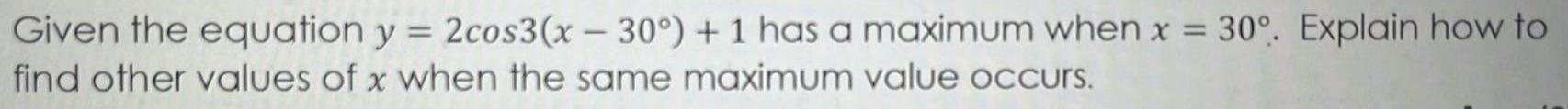Geometry
Solution of triangles
Given the equation y = 2cos3(x - 30°) + 1 has a maximum when x = 30°. Explain how to find other values of x when the same maximum value occurs.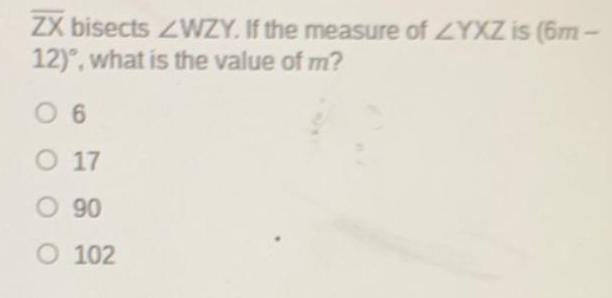Geometry
Solution of triangles
ZX bisects WZY. If the measure of YXZ is (6m-12), what is the value of m? 6 17 90 102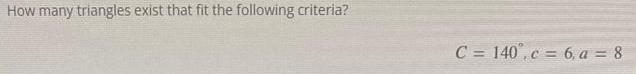Geometry
Solution of triangles
How many triangles exist that fit the following criteria? C= 140, c = 6, a = 8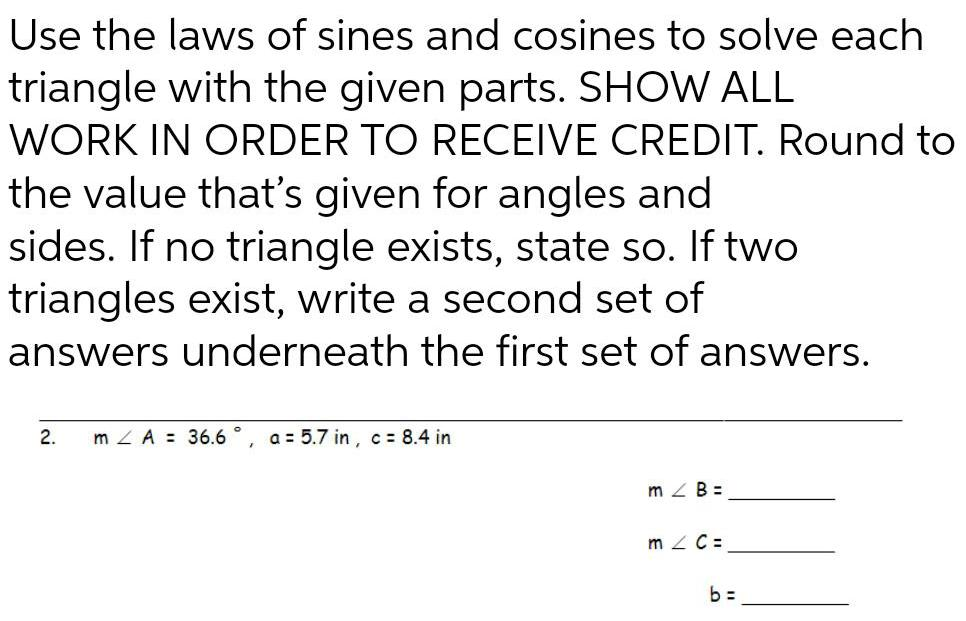Geometry
Solution of triangles
Use the laws of sines and cosines to solve each triangle with the given parts. SHOW ALL WORK IN ORDER TO RECEIVE CREDIT. Round to the value that's given for angles and sides. If no triangle exists, state so. If two triangles exist, write a second set of answers underneath the first set of answers. ∠A = 36.6 ° a = 5.7 in, c= 8.4 in m∠B= m ∠ C= b=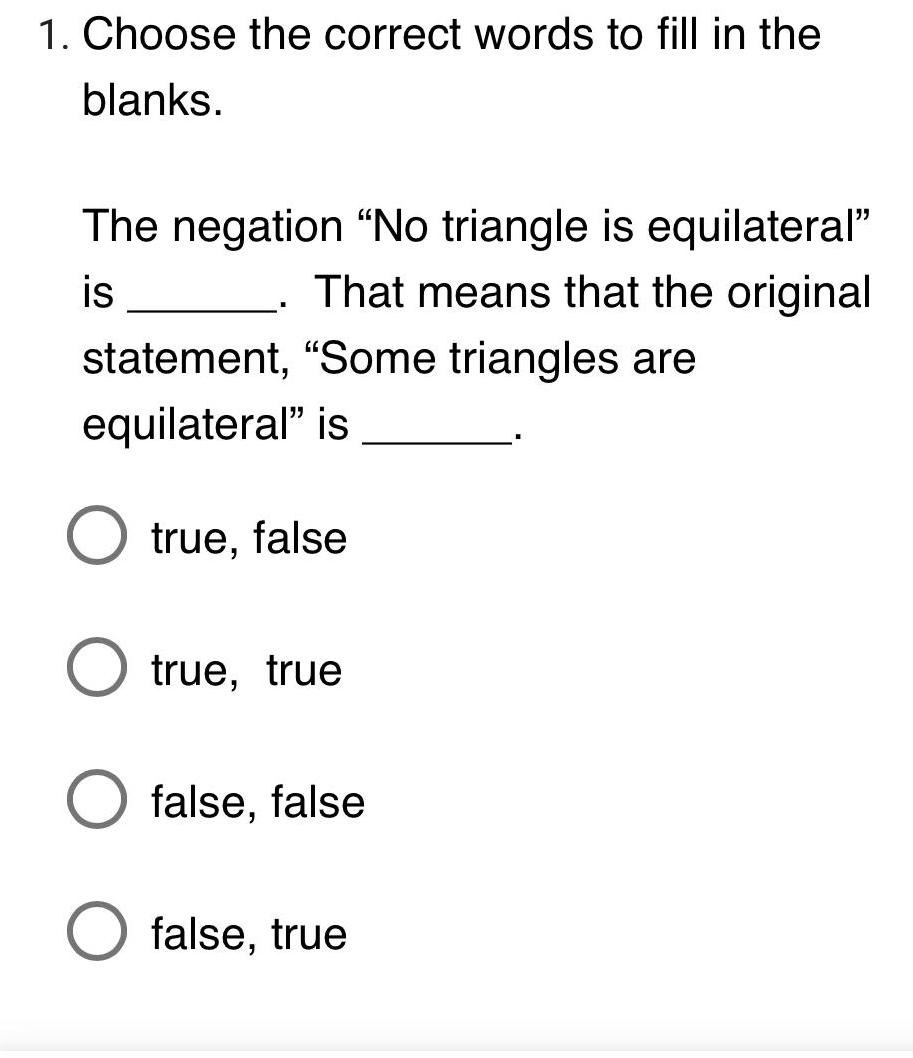Geometry
Solution of triangles
1. Choose the correct words to fill in the blanks. The negation "No triangle is equilateral" is.That means that the original statement, "Some triangles are equilateral" is true, false true, true false, false false, true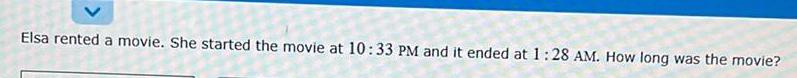Geometry
Solution of triangles
Elsa rented a movie. She started the movie at 10:33 PM and it ended at 1:28 AM. How long was the movie?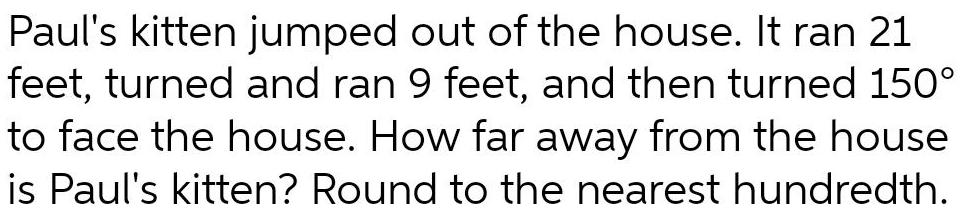Geometry
Solution of triangles
Paul's kitten jumped out of the house. It ran 21 feet, turned and ran 9 feet, and then turned 150° to face the house. How far away from the house is Paul's kitten? Round to the nearest hundredth.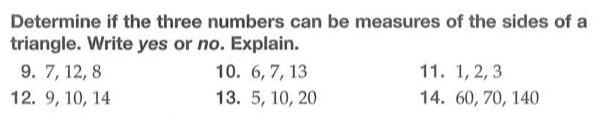Geometry
Solution of triangles
Determine if the three numbers can be measures of the sides of a triangle. Write yes or no. Explain. 6, 7, 13 5, 10, 20 7, 12, 8 9, 10, 14 1, 2, 3 60, 70, 140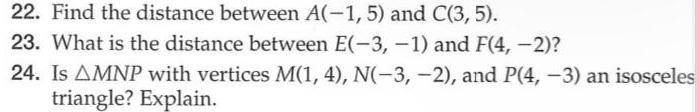Geometry
Solution of triangles
Find the distance between A(-1, 5) and C(3, 5). What is the distance between E(-3,-1) and F(4, -2)? Is AMNP with vertices M(1, 4), N(-3, -2), and P(4, -3) an isosceles triangle? Explain.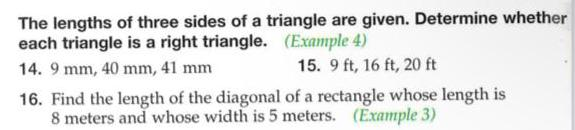Geometry
Solution of triangles
The lengths of three sides of a triangle are given. Determine whether Each triangle is a right triangle. 9 mm, 40 mm, 41 mm 19 ft, 16 ft, 20 ft Find the length of the diagonal of a rectangle whose length is 8 meters and whose width is 5 meters. (Example 3)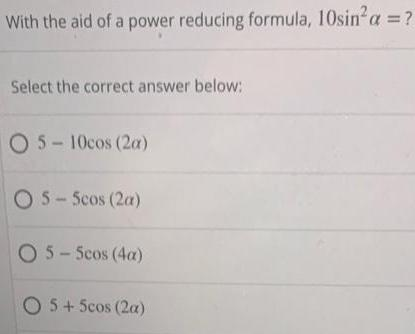Geometry
Solution of triangles
With the aid of a power reducing formula, 10sin²a = ? Select the correct answer below: 5-10cos (2a) 5-5cos (2a) 5-5cos (4a) 5+5cos (2a)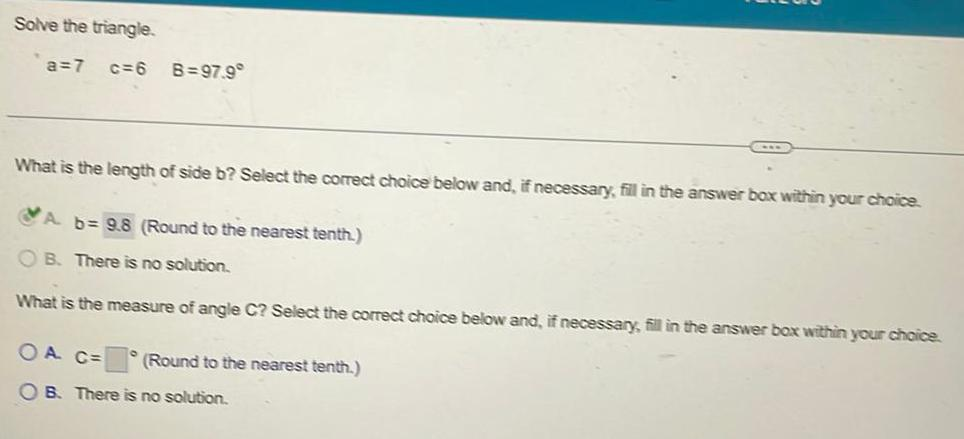Geometry
Solution of triangles
Solve the triangle. a=7 c=6 B=97.9° What is the length of side b? Select the correct choice below and, if necessary, fill in the answer box within your choice. A. b= (Round to the nearest tenth.) B. There is no solution. What is the measure of angle C? Select the correct choice below and, if necessary, fill in the answer box within your choice. A C= B. There is no solution. (Round to the nearest tenth.)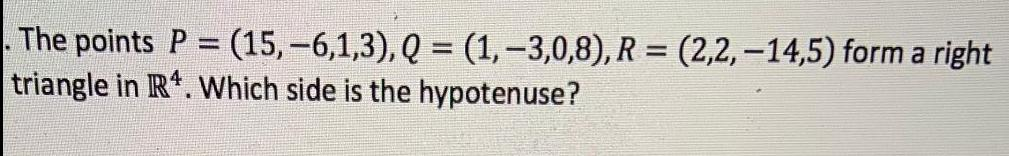Geometry
Solution of triangles
The points P = (15, −6,1,3), Q = (1, −3,0,8), R = (2,2, -14,5) form a right triangle in R^4. Which side is the hypotenuse?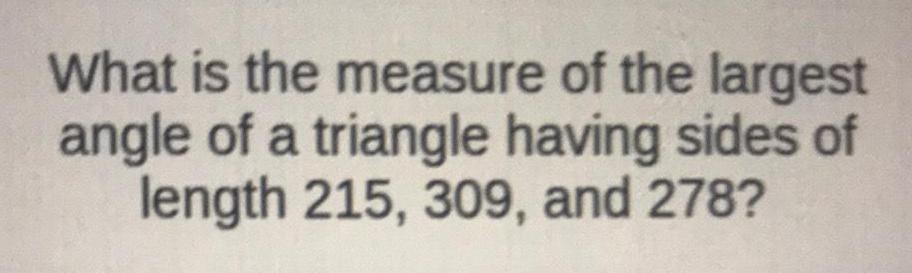Geometry
Solution of triangles
What is the measure of the largest angle of a triangle having sides of length 215, 309, and 278?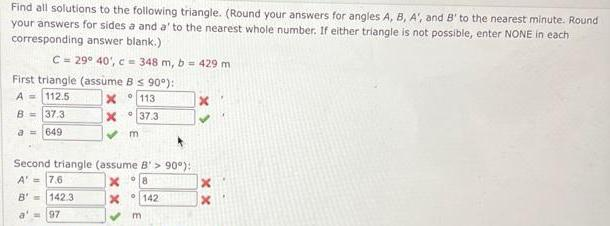Geometry
Solution of triangles
Find all solutions to the following triangle. (Round your answers for angles A, B, A', and B' to the nearest minute. Round your answers for sides a and a' to the nearest whole number. If either triangle is not possible, enter NONE in each corresponding answer blank.) C= 29° 40', c = 348 m, b = 429 m First triangle (assume B ≤ 90°): A = B = a = Second triangle (assume B'> 90°): A' = B' = a' =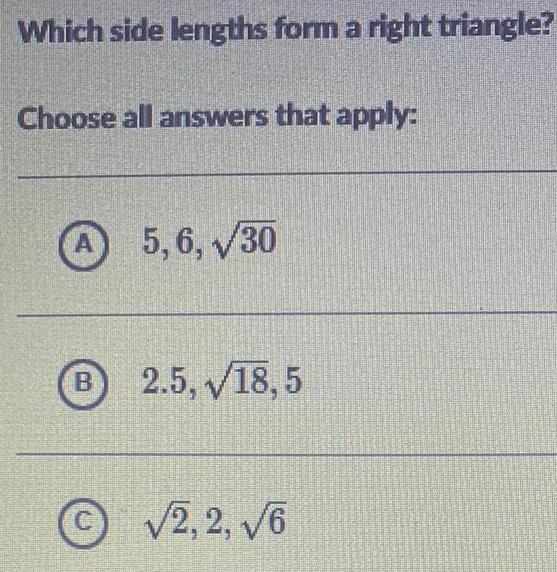Geometry
Solution of triangles
Which side lengths form a right triangle? Choose all answers that apply: A. 5,6,√30 B. 2.5, 18,5 C. √2,2, √6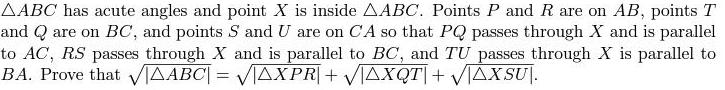Geometry
Solution of triangles
AABC has acute angles and point X is inside AABC. Points P and R are on AB, points T and Q are on BC, and points S and U are on CA so that PQ passes through X and is parallel to AC, RS passes through X and is parallel to BC, and TU passes through X is parallel to BA. Prove that √|√△ABC|=√△XPR+√△XQT+√△XSUI.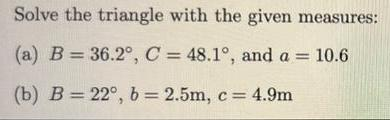Geometry
Solution of triangles
Solve the triangle with the given measures: (a) B = 36.2°, C = 48.1°, and a = 10.6 (b) B= 22°, b = 2.5m, c = 4.9m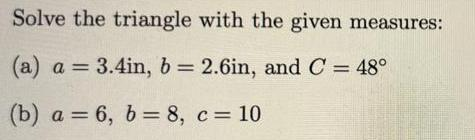Geometry
Solution of triangles
Solve the triangle with the given measures: (a) a = 3.4in, b = 2.6in, and C = 48° (b) a = 6, b = 8, c = 10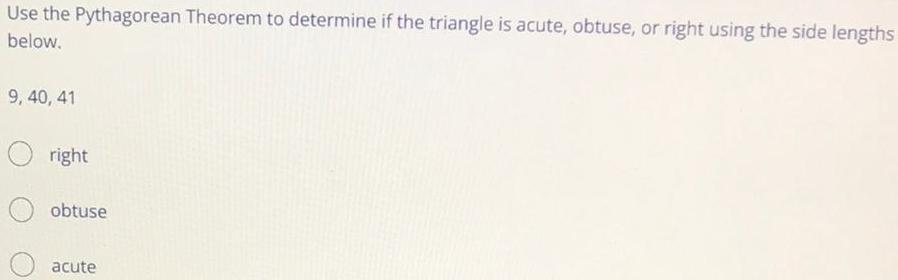Geometry
Solution of triangles
Use the Pythagorean Theorem to determine if the triangle is acute, obtuse, or right using the side lengths below. 9, 40, 41 right obtuse acute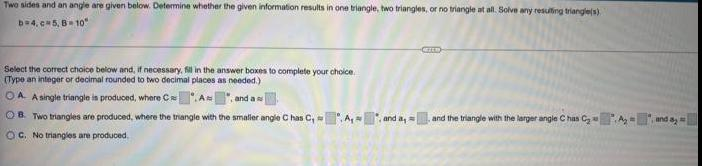Geometry
Solution of triangles
Two sides and an angle are given below. Determine whether the given information results in one triangle, two triangles, or no triangle at all. Solve any resulting triangle(s) b=4.c 5, B 10" Select the correct choice below and, if necessary, fill in the answer boxes to complete your choice. (Type an integer or decimal rounded to two decimal places as needed.) OAA single triangle is produced, where CAN and a OB. Two triangles are produced, where the triangle with the smaller angle Chas C, A, and a and the triangle with the larger angle Chas C₂______and a₂ OC. No triangles are produced.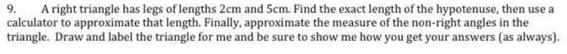Geometry
Solution of triangles
A right triangle has legs of lengths 2cm and 5cm. Find the exact length of the hypotenuse, then use a calculator to approximate that length. Finally, approximate the measure of the non-right angles in the triangle. Draw and label the triangle for me and be sure to show me how you get your answers (as always).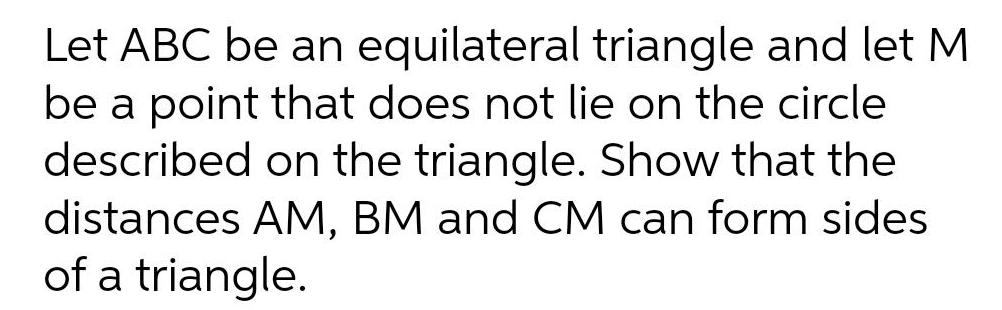Geometry
Solution of triangles
Let ABC be an equilateral triangle and let M be a point that does not lie on the circle described on the triangle. Show that the distances AM, BM and CM can form sides of a triangle.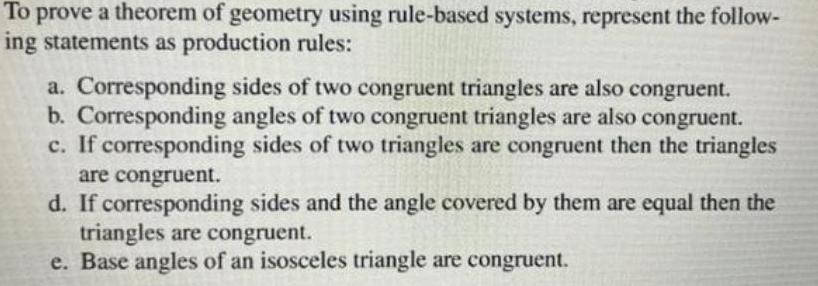Geometry
Solution of triangles
To prove a theorem of geometry using rule-based systems, represent the following statements as production rules: a. Corresponding sides of two congruent triangles are also congruent. b. Corresponding angles of two congruent triangles are also congruent. c. If corresponding sides of two triangles are congruent then the triangles are congruent. d. If corresponding sides and the angle covered by them are equal then the triangles are congruent. e. Base angles of an isosceles triangle are congruent.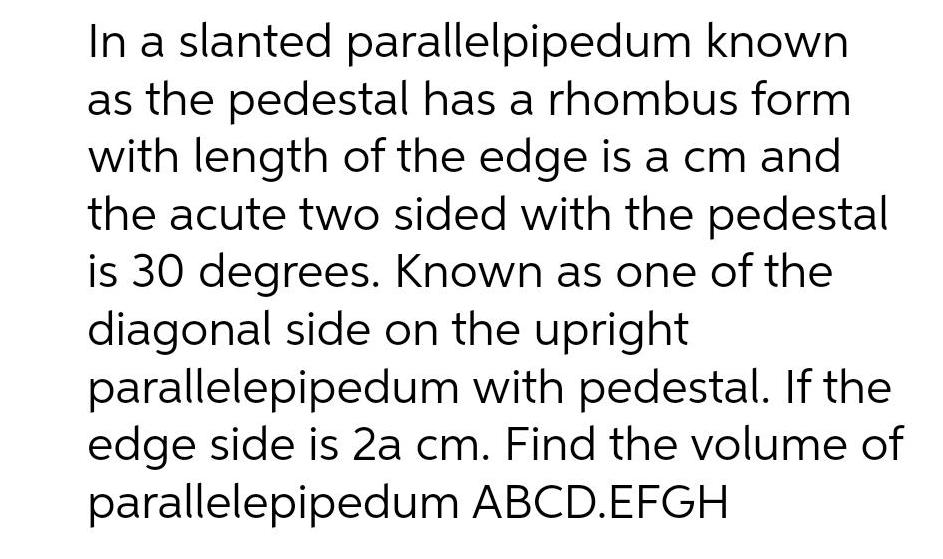Geometry
Solution of triangles
In a slanted parallelpipedum known as the pedestal has a rhombus form with length of the edge is a cm and the acute two sided with the pedestal is 30 degrees. Known as one of the diagonal side on the upright parallelepipedum with pedestal. If the edge side is 2a cm. Find the volume of parallelepipedum ABCD.EFGH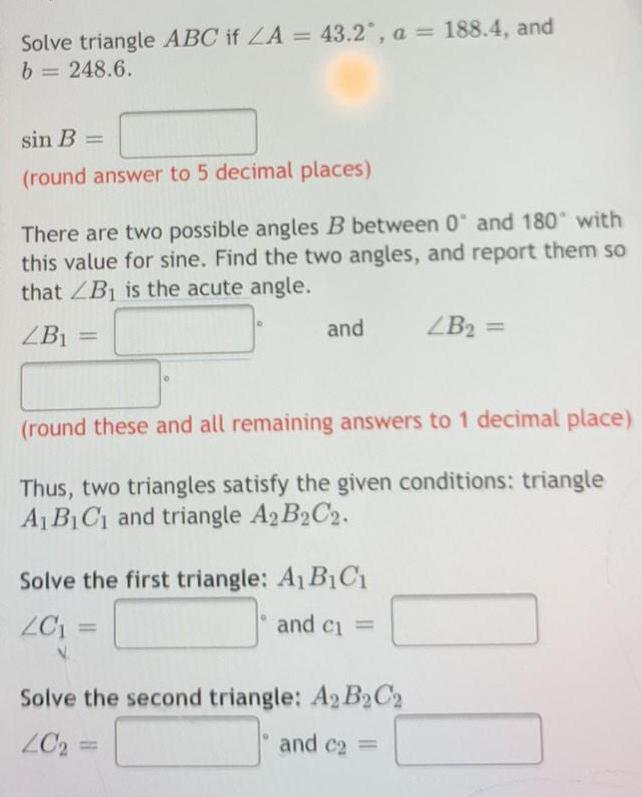Geometry
Solution of triangles
Solve triangle ABC if ^A = 43.2°, a = 188.4, and b = 248.6. sin B = (round answer to 5 decimal places) There are two possible angles B between 0 and 180" with this value for sine. Find the two angles, and report them so that ^B₁ is the acute angle. ^B1= and ^B₂ = (round these and all remaining answers to 1 decimal place) Thus, two triangles satisfy the given conditions: triangle A₁B₁C₁ and triangle A2B2C2. Solve the first triangle: A₁B₁C₁ ^C₁ and c₁ = Solve the second triangle: A₂ B₂C2 ^C₂= and c2 =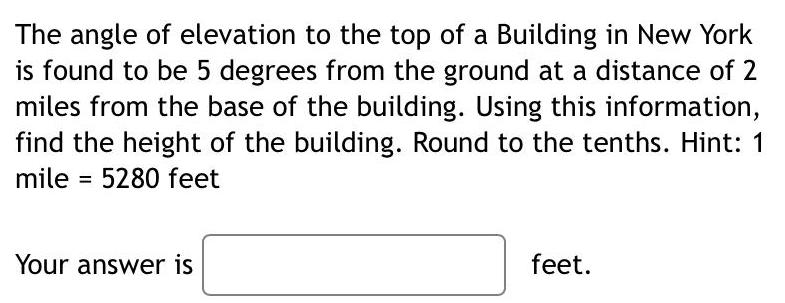Geometry
Solution of triangles
The angle of elevation to the top of a Building in New Yorkis found to be 5 degrees from the ground at a distance of 2miles from the base of the building. Using this information,find the height of the building. Round to the tenths.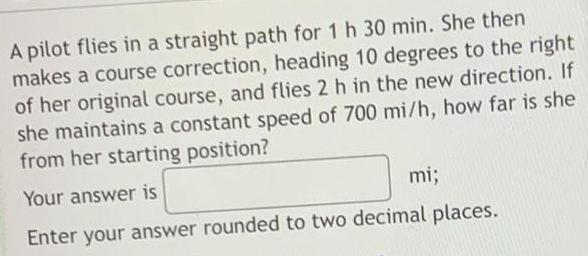Geometry
Solution of triangles
A pilot flies in a straight path for 1 h 30 min. She thenmakes a course correction, heading 10 degrees to the rightof her original course, and flies 2 h in the new direction. Ifshe maintains a constant speed of 700 mi/h, how far is shefrom her starting position? Your answer is_______Enter your answer rounded to two decimal places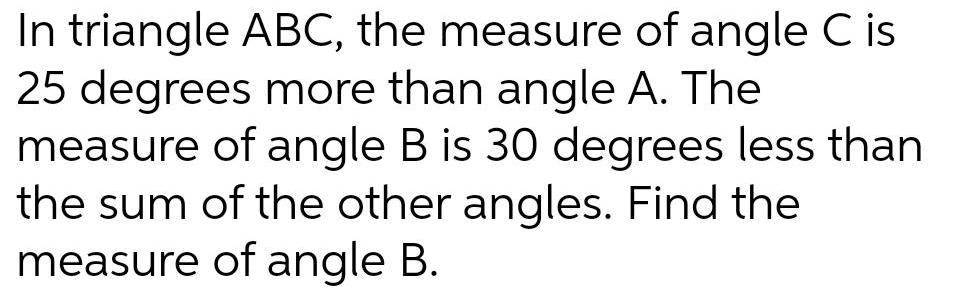Geometry
Solution of triangles
In triangle ABC, the measure of angle C is 25 degrees more than angle A. The measure of angle B is 30 degrees less than the sum of the other angles. Find the measure of angle B.